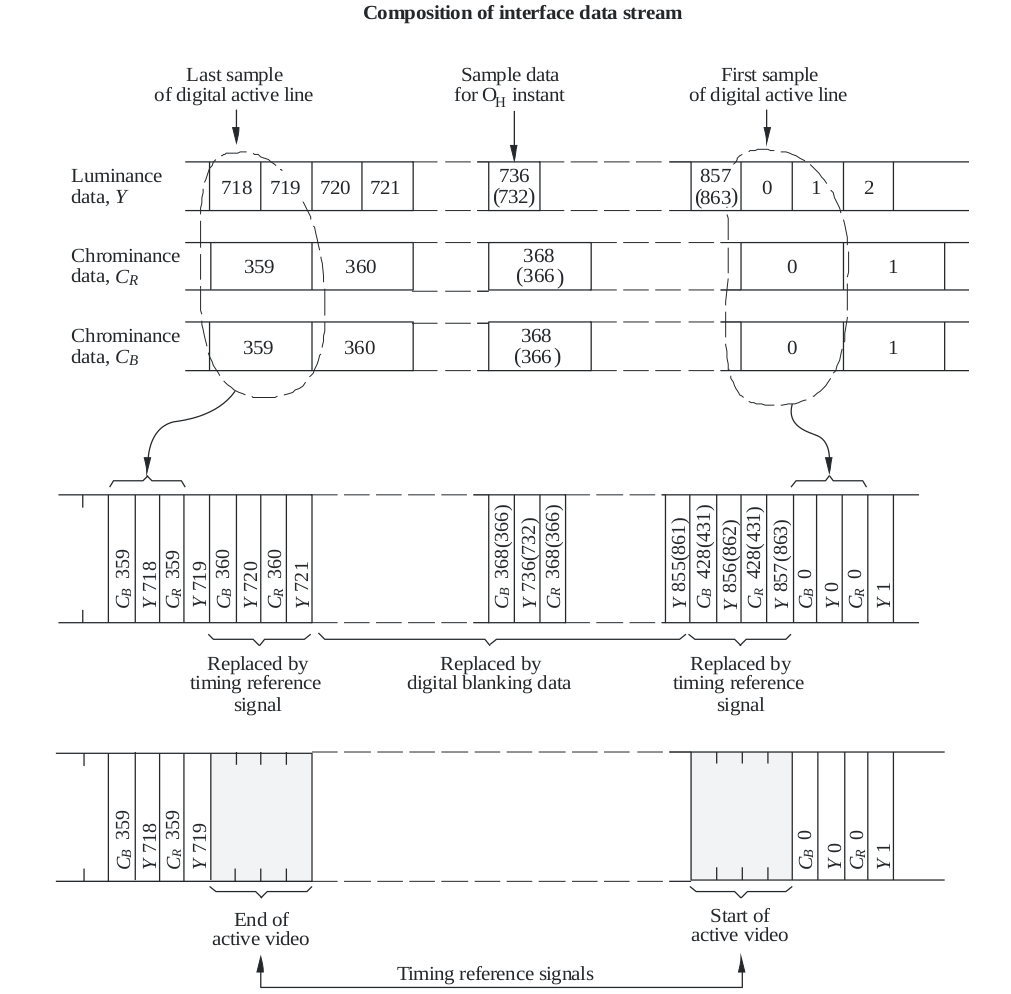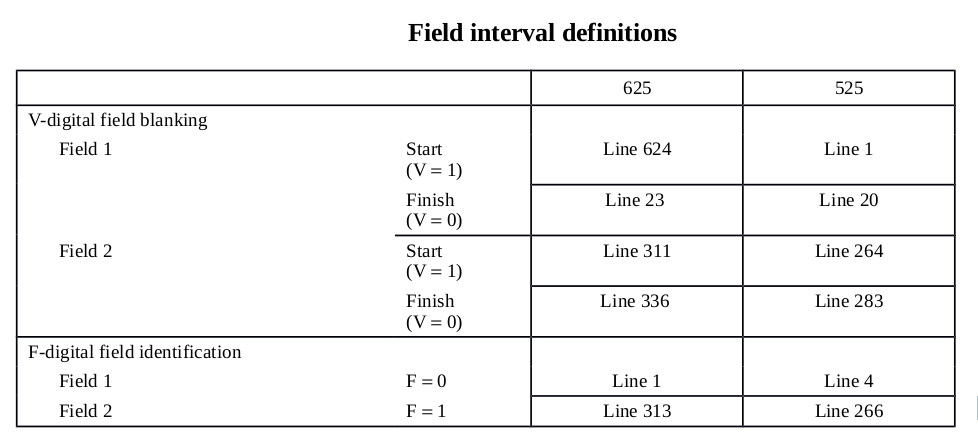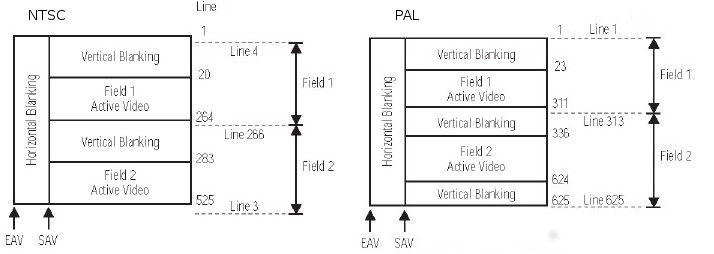ITU-R — International Telecommunication Union Radiocommunication

ITU-R Bt.656是标清数字视频信号传输标准

# 帧场的概念

NTSC制共525行扫描线，每秒60场图像. PAL制为625行扫描线，每秒50场图像.

PAL制NTSC制的技术指标:

PAL 50Hz 25Hz 15625Hz 625 25 x 2 576
NTSC 29.97 x 2 Hz 29.97 Hz 15734.25Hz 525 20 x 2 486

# 数字信号格式

• 视频信号(video signals)
• 数字消隐数据(digital blanking data)
• 定时基准信号(time reference signals)
• 辅助信号(ancillary signals)

# 定时基准信号

SAV(start of active video) 每个视频数据块的开始(at the beginning of each video data block)
EAV(end of active video) 每个视频数据块的结尾10bit形式: 3FF 000 000 XYZ
8bit形式: FF 00 00 XY

bit9 (MSB) bit8 bit7 bit6 bit5 bit4 bit3 bit2 bit1 bit0
1 F V H P3 P2 P1 P0 0 0

bit7 (MSB) bit6 bit5 bit4 bit3 bit2 bit1 bit0
1 F V H P3 P2 P1 P0

F 场同步信号:

- F = 0 表示field1
- F = 1 表示field2


V 垂直同步信号:

- V = 0 表示接下来的数据为有效图像数据.
- V = 1 表示接下来的数据为消隐区数据.


H 水平同步信号:

- H = 0 表示SAV
- H = 1 表示EAV


P0 P1 P2 P4 是保护位(protection bits)，用来校验F V H, 校验规则如下:

- P3=V(XOR)H；P2=F(XOR)H；P1=F(XOR)V；P0=F(XOR)V(XOR)H


F V的定义见下表:P3 P2 P1 P0 与 F V H的关系见下表:

$$\begin{array}{ccc|cccc|} \text{ F } & \text{ V } & \text{ H } & \text{P3} & \text{P2} & \text{P1} & \text{P0} \\ \hline 0 & 0 & 0 & 0 & 0 & 0 & 0 \\ 0 & 0 & 1 & 1 & 1 & 0 & 1 \\ 0 & 1 & 0 & 1 & 0 & 1 & 1 \\ 0 & 1 & 1 & 0 & 1 & 1 & 0 \\ 1 & 0 & 0 & 0 & 1 & 1 & 1 \\ 1 & 0 & 1 & 1 & 0 & 1 & 0 \\ 1 & 1 & 0 & 1 & 1 & 0 & 0 \\ 1 & 1 & 1 & 0 & 0 & 0 & 1 \\ \end{array}$$

# BT656行场帧的构成

- 场 = First Vertical Blanking + Active Video lines + Second Vertical Blanking
-
- 对于顶场(Top Field),    Active Video 就是一帧图像的所有偶数行
- 对于底场(Bottom Field), Active Video 就是一帧图像的所有奇数行
- NTSC先传输bottom field, PAL先传输top field


- 行 = 结束码(EAV) + 水平消隐(Horizontal Blanking) + 起始码(SAV) + 有效数据(Active video data)


BT656 行场帧构成的示意图:$$\begin{array}{cc} \begin{array}{c|ccccc} \text{行数} & \text{ F } & \text{ V } & \text{EAV(H=1)} & \text{SAV(H=0)} \\ \hline \text{4~19} & 0 & 1 & \text{(1011 0110) 0xB6} & \text{(1010 1011) 0xAB} \\ \text{20~263} & 0 & 0 & \text{(1001 1101) 0x9D} & \text{(1000 0000) 0x80} \\ \text{264~265} & 0 & 1 & \text{(1011 0110) 0xB6} & \text{(1010 1011) 0xAB} \\ \hline \text{266~282} & 1 & 1 & \text{(1111 0001) 0xF1} & \text{(1110 1100) 0xEC} \\ \text{283~525} & 1 & 0 & \text{(1101 1010) 0xDA} & \text{(1100 0111) 0xC7} \\ \text{1~3} & 1 & 1 & \text{(1111 0001) 0xF1} & \text{(1110 1100) 0xEC} \\ \end{array} \qquad \qquad \begin{array}{c|cccc} \text{行数} & \text{ F } & \text{ V } & \text{EAV(H=1)} & \text{SAV(H=0)} \\ \hline \text{1~22} & 0 & 1 & \text{(1011 0110) 0xB6} & \text{(1010 1011) 0xAB} \\ \text{23~310} & 0 & 0 & \text{(1001 1101) 0x9D} & \text{(1000 0000) 0x80} \\ \text{311~312} & 0 & 1 & \text{(1011 0110) 0xB6} & \text{(1010 1011) 0xAB} \\ \hline \text{313~335} & 1 & 1 & \text{(1011 0110) 0xB6} & \text{(1010 1011) 0xAB} \\ \text{336~623} & 1 & 0 & \text{(1001 1101) 0x9D} & \text{(1000 0000) 0x80} \\ \text{624~625} & 1 & 1 & \text{(1011 0110) 0xB6} & \text{(1010 1011) 0xAB} \\ \end{array} \end{array}$$

一帧有625行,其中顶场有效数据和底场有效数据分别为288行.因为PAL制的SDTV或者D1的分辨率为720x576



Field1: 16行(first vertical blanking) + 244行 + 2行(second vertical blanking)
Field2: 17行(first vertical blanking) + 243行 + 3行(second vertical blanking)